Next: Reality and the Role Up: Quantum Theory Without Observers Previous: Spontaneous Localization

# Bohmian Mechanics

In the pilot-wave (or Bohmian) approach, quantum theory is fundamentally about the behavior of particles, described by their positions--or fields, described by field configurations, or strings, described by string configurations--and only secondarily about wave functions. In this approach the wave function, obeying Schrödinger's equation, does not provide a complete description or representation of a quantum system. Instead, the wave function choreographs or governs the motion of the more fundamental variables.

Bohmian mechanics (or the de Broglie-Bohm theory) is the minimal completion of Schrödinger's equation, for a nonrelativistic system of particles, to a theory describing a genuine motion of particles. For Bohmian mechanics the state of the system is described by its wave functiontogether with the configuration Q defined by the positions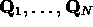of its particles. The theory is then defined by two evolution equations: Schrödinger's equation for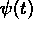and a first-order evolution equation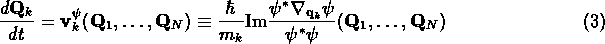for Q(t), the simplest first-order evolution equation for the positions of the particles that is compatible with the Galilean (and time-reversal) covariance of the Schrödinger evolution [24, pages 852-854,]. Here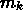is the mass of the k-th particle. (Ifis spinor-valued, the products in numerator and denominator should be understood as scalar products. If external magnetic fields are present, the gradient should be understood as the covariant derivative, involving the vector potential.) This deterministic theory of particles in motion completely accounts for all the phenomena of nonrelativistic quantum mechanics, from interference effects to spectral lines [23, pages 175-178,] to spin [25, page 10 of ,], and it does so in a completely ordinary manner.

Note that given an initial wave function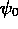, the full Bohmian trajectory Q(t) is determined by the initial configuration . Thus, given any probability distribution for the initial configuration, Bohmian mechanics defines a probability distribution for the full trajectory. Moreover, since the right hand side of (3) is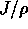, where J is the quantum probability current and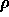is the quantum probability density, it follows from the quantum continuity equationthat if the distribution of the configuration Q is given byat some time (say the initial time) this will be true at all times. Thus Bohmian mechanics provides us with probabilities for completely fine-grained configurational histories that are consistent with the quantum mechanical probabilities for configurations, including the positions of instrument pointers, at single times.

The pilot-wave approach to quantum theory was initiated, even before the discovery of quantum mechanics itself, by Einstein, who hoped that interference phenomena involving particle-like photons could be explained if the motion of the photons were somehow guided by the electromagnetic field--which would thus play the role of what he called a Führungsfeld or guiding field [26, page 262,]. While the notion of the electromagnetic field as guiding field turned out to be rather problematical, the possibility that for a system of electrons the wave function might play this role, of guiding field or pilot wave, was explored by Max Born in his early paper founding quantum scattering theory --a suggestion to which Heisenberg was profoundly unsympathetic.

By 1927, an equation of particle motion equivalent to (3) for a scalar wave function had been written down by Louis de Broglie [28, page 119,], who explained at the 1927 Solvay Congress how this motion could account for quantum interference phenomena. However, de Broglie badly failed to respond adequately to an objection of Wolfgang Pauli [30, pages 280-282,] concerning inelastic scattering, no doubt making a rather poor impression on the illustrious audience gathered for the occasion (see Figure 7).

Born and De Broglie very quickly abandoned the pilot-wave approach and became enthusiastic supporters of the rapidly developing consensus in favor of the Copenhagen interpretation. Bohmian mechanics was rediscovered in 1952 by David Bohm, the first person genuinely to understand its significance and implications. (Unfortunately, Bohm's formulation involved unnecessary complications and could not deal efficiently with spin. In particular, Bohm's invocation of the ``quantum potential'' made his theory seem artificial and obscured its essential structure. For more on this, see .) Its principal advocate during the past three decades was Bell . Impelled by the evident nonlocality of Bohmian mechanics, Bell  established, using the ``no-hidden-variables theorem'' based on his famous inequality, that this feature was unavoidable by any serious theory accounting for the quantum predictions.

The possibility of a deterministic reformulation of quantum theory such as Bohmian mechanics has been regarded by almost all luminaries of quantum physics as having been conclusively refuted. For several decades this refutation was believed to have been provided by the 1932 no-hidden-variables proof of John von Neumann , despite the fact that, according to Bell , von Neumann's assumptions are so unreasonable that ``the proof of von Neumann is not merely false but foolish!'' While some physicists continue to rely on von Neumann's proof, it is interesting to note that in recent years it is more common to find physicists citing Bell's no-hidden-variables theorem as the basis of this refutation--thus failing to appreciate that what Bell demonstrated with his theorem was not the impossibility of Bohmian mechanics but rather that its most radical implication, namely nonlocality, was intrinsic to quantum theory itself.

According to Richard Feynman, the two-slit experiment for electrons is [34, page 37-2,] ``a phenomenon which is impossible, absolutely impossible, to explain in any classical way, and which has in it the heart of quantum mechanics. In reality it contains the only mystery.'' This experiment [35, page 130,] ``has been designed to contain all of the mystery of quantum mechanics, to put you up against the paradoxes and mysteries and peculiarities of nature one hundred per cent.'' As to the question [35, page 145,], ``How does it really work? What machinery is actually producing this thing? Nobody knows any machinery. Nobody can give you a deeper explanation of this phenomenon than I have given; that is, a description of it.''

But Bohmian mechanics is just such a deeper explanation (as is SL, of which, however, Feynman could not have been aware). It resolves the dilemma of the appearance, in one and the same phenomenon, of both particle and wave properties in a rather straightforward manner: Bohmian mechanics is a theory of motion describing a particle (or particles) guided by a wave. In Figure 1 we have a family of Bohmian trajectories for the two-slit experiment. While each trajectory passes through but one of the slits, the wave passes through both; the interference profile that therefore develops in the wave generates a similar pattern in the trajectories guided by this wave.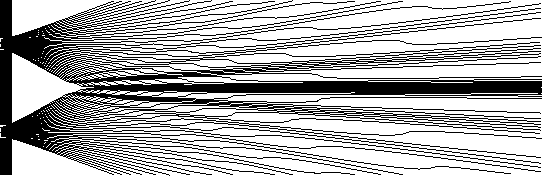Figure 1: An ensemble of trajectories for the two-slit experiment, uniform in the slits. (Drawn by G. Bauer from .)

Compare Feynman's presentation with that of Bell [1, page 191,]:

Is it not clear from the smallness of the scintillation on the screen that we have to do with a particle? And is it not clear, from the diffraction and interference patterns, that the motion of the particle is directed by a wave? De Broglie showed in detail how the motion of a particle, passing through just one of two holes in screen, could be influenced by waves propagating through both holes. And so influenced that the particle does not go where the waves cancel out, but is attracted to where they cooperate. This idea seems to me so natural and simple, to resolve the wave-particle dilemma in such a clear and ordinary way, that it is a great mystery to me that it was so generally ignored. (Bell 1986)

Nonetheless, it would appear that inasmuch as orthodox quantum theory supplies us with probabilities not merely for positions but for a huge class of quantum observables, it is a much richer theory than Bohmian mechanics, which seems exclusively concerned with positions. Appearances are, however, misleading. In this regard, as with so much else in the foundations of quantum mechanics, the crucial observation has been made by Bell [1, page 166,]:

...in physics the only observations we must consider are position observations, if only the positions of instrument pointers. It is a great merit of the de Broglie-Bohm picture to force us to consider this fact. If you make axioms, rather than definitions and theorems, about the `measurement' of anything else, then you commit redundancy and risk inconsistency. (Bell 1982)

Bell's point here is well taken: the usual measurement postulates of quantum theory, including collapse of the wave function and probabilities for measurement results given by the absolute square of probability amplitudes, emerge  as soon as we take seriously the equations of Bohmian mechanics and what they describe--provided that the initial configuration of a system is random, with probability distribution given by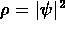. Moreover, Detlef Dürr, Nino Zanghì, and I have shown  how probabilities for positions given byemerge naturally from an analysis of ``equilibrium'' for the deterministic dynamical system defined by Bohmian mechanics, in much the same way that the Maxwellian velocity distribution emerges from an analysis of classical thermodynamic equilibrium. Thus with Bohmian mechanics the statistical description in quantum theory indeed takes, as Einstein anticipated, ``an approximately analogous position to the statistical mechanics within the framework of classical mechanics.''Next: Reality and the Role Up: Quantum Theory Without Observers Previous: Spontaneous Localization

Shelly Goldstein
Wed Aug 13 17:22:41 EDT 1997